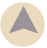# Percentage of Two Numbers Calculator

This calculator determines the percentage of one number to another number. For example, if the first number is 100 and the second number is 1000, the percentage is 10%, but if the second number is 10,000, the percentage is 1%.

## Calculate percent of one number in relation to second

What percent is
Of:

Example: What percentage is 10 of 100? (Answer 10 percent)

### More Percentage Calculators